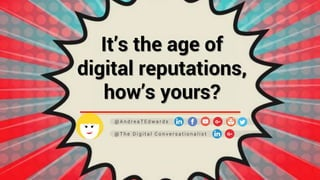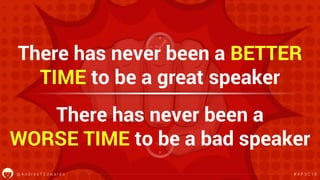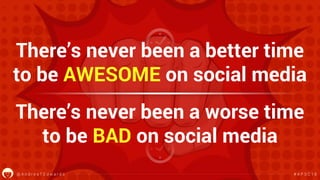Successfully reported this slideshow.

# It's the age of digital reputations, how's yours?×

# It's the age of digital reputations, how's yours?

A presentation for professional speakers at the Asia Professional Speakers Convention 2018 in Singapore. Focused on three take-aways - be world-class, be of service, and join the Giving Economy. The emphasis is on all of us owning the digital stage, with the same energy, commitment and passion as we give to the physical stage.

A presentation for professional speakers at the Asia Professional Speakers Convention 2018 in Singapore. Focused on three take-aways - be world-class, be of service, and join the Giving Economy. The emphasis is on all of us owning the digital stage, with the same energy, commitment and passion as we give to the physical stage.

### It's the age of digital reputations, how's yours?

1. 1. @ A n d r e a T E d w a r d s # A P S C 1 8 It’s the age of digital reputations, how’s yours? @ A n d r e a T E d w a r d s @ T h e D i g i t a l C o n v e r s a t i o n a l i s t
2. 2. @ A n d r e a T E d w a r d s # A P S C 1 8 There has never been a BETTER TIME to be a great speaker There has never been a WORSE TIME to be a bad speaker @ A n d r e a T E d w a r d s # A P S C 1 8
3. 3. @ A n d r e a T E d w a r d s # A P S C 1 8 There’s never been a better time to be AWESOME on social media There’s never been a worse time to be BAD on social media @ A n d r e a T E d w a r d s # A P S C 1 8
4. 4. @ A n d r e a T E d w a r d s # A P S C 1 8 This is our digital stage
5. 5. @ A n d r e a T E d w a r d s # A P S C 1 8 Passion Enthusiasm Energy Commitment @ A n d r e a T E d w a r d s # A P S C 1 8
6. 6. @ A n d r e a T E d w a r d s # A P S C 1 8 FOMO @ A n d r e a T E d w a r d s # A P S C 1 8
7. 7. @ A n d r e a T E d w a r d s # A P S C 1 8@ A n d r e a T E d w a r d s # A P S C 1 8
8. 8. @ A n d r e a T E d w a r d s # A P S C 1 8 Matthew Crawford Attention is a resource a person has only so much of it ATTENTION ECONOMY @ A n d r e a T E d w a r d s
9. 9. @ A n d r e a T E d w a r d s # A P S C 1 8@ A n d r e a T E d w a r d s
10. 10. @ A n d r e a T E d w a r d s # A P S C 1 8 Look at the numbers… 3 Billion On Social Media 1 Million New active mobile social users (Source: We Are Social) Enough blogs published to fill Time Magazine for 770 years EVERY SINGLE DAY @ A n d r e a T E d w a r d s # A P S C 1 8
11. 11. @ A n d r e a T E d w a r d s # A P S C 1 8
12. 12. @ A n d r e a T E d w a r d s # A P S C 1 8 CONTENT SHOCK… 01 Data asphyxiation - William van Winkle 02 03 04 05 06 Data smog - David Shenk Information fatigue syndrome - David Lewis Cognitive overload - Eric Schmidt Time famine - Leslie Perlow Infobesity or infoxication - Wikipedia @ A n d r e a T E d w a r d s # A P S C 1 8
13. 13. @ A n d r e a T E d w a r d s # A P S C 1 8
14. 14. @ A n d r e a T E d w a r d s # A P S C 1 8 BIG TIME?
15. 15. @ A n d r e a T E d w a r d s # A P S C 1 8 SERVICE MINDSET @ A n d r e a T E d w a r d s # A P S C 1 8
16. 16. @ A n d r e a T E d w a r d s # A P S C 1 8
17. 17. @ A n d r e a T E d w a r d s # A P S C 1 8 Why not bigger? Not good enough? No lucky break? Or not enough of a hustler?
18. 18. @ A n d r e a T E d w a r d s # A P S C 1 8 IN THE BUSINESS OF EARNING TIME… @ A n d r e a T E d w a r d s # A P S C 1 8
19. 19. @ A n d r e a T E d w a r d s # A P S C 1 8 Start with YOUR WHY @ A n d r e a T E d w a r d s # A P S C 1 8
20. 20. @ A n d r e a T E d w a r d s # A P S C 1 8 My Why... I’m changing the world for the better @ A n d r e a T E d w a r d s # A P S C 1 8
21. 21. @ A n d r e a T E d w a r d s # A P S C 1 8 Meaning What’s your cause? Powerful @ A n d r e a T E d w a r d s # A P S C 1 8 A cause to support
22. 22. @ A n d r e a T E d w a r d s # A P S C 1 8 Business leaders 100% Do NOT like the social noise @ A n d r e a T E d w a r d s # A P S C 1 8
23. 23. @ A n d r e a T E d w a r d s # A P S C 1 8 We can’t contribute to the noise
24. 24. @ A n d r e a T E d w a r d s # A P S C 1 8 Pay Attention to your field @ A n d r e a T E d w a r d s # A P S C 1 8
25. 25. @ A n d r e a T E d w a r d s # A P S C 1 8 Track your industry Go deep in your area Who’s doing great? What bad looks like? @ A n d r e a T E d w a r d s # A P S C 1 8
26. 26. @ A n d r e a T E d w a r d s # A P S C 1 8 Is it World Class? @ A n d r e a T E d w a r d s @ T h e D i g i t a l C o n v e r s a t i o n a l i s t
27. 27. @ A n d r e a T E d w a r d s # A P S C 1 8
28. 28. @ A n d r e a T E d w a r d s # A P S C 1 8 Create conversations Worth investing in Respond, respond, respond @ A n d r e a T E d w a r d s # A P S C 1 8 Keeps it top of feed
29. 29. @ A n d r e a T E d w a r d s # A P S C 1 8
30. 30. @ A n d r e a T E d w a r d s # A P S C 1 8 I’m sorry but… Not genuine No magic Diminishes value Outsource a speaking opp? No one should outsource
31. 31. @ A n d r e a T E d w a r d s # A P S C 1 8@ A n d r e a T E d w a r d s # A P S C 1 8
32. 32. @ A n d r e a T E d w a r d s # A P S C 1 8 Inspiration to make your day, but sometimes when you’re in a bad mood and someone shares a positive quote, you just want to smack them in the head Quote from a Dead White Man
33. 33. @ A n d r e a T E d w a r d s # A P S C 1 8 It’s an Act of Service @ A n d r e a T E d w a r d s @ T h e D i g i t a l C o n v e r s a t i o n a l i s t
34. 34. @ A n d r e a T E d w a r d s # A P S C 1 8@ A n d r e a T E d w a r d s # A P S C 1 8
35. 35. @ A n d r e a T E d w a r d s # A P S C 1 8 How do you build trust? Deliver value01 Better, richer or deeper 02 Making your mum happy? 03 @ A n d r e a T E d w a r d s # A P S C 1 8
36. 36. @ A n d r e a T E d w a r d s # A P S C 1 8 Own your digital stage Tie it to a mission @ A n d r e a T E d w a r d s # A P S C 1 8
37. 37. @ A n d r e a T E d w a r d s # A P S C 1 8 Be of service It’s not about you. It’s never been about you. @ A n d r e a T E d w a r d s # A P S C 1 8
38. 38. @ A n d r e a T E d w a r d s # A P S C 1 8@ A n d r e a T E d w a r d s # A P S C 1 8
39. 39. @ A n d r e a T E d w a r d s # A P S C 1 8@ A n d r e a T E d w a r d s One-stop-shop in content marketing, employee advocacy, social leadership Takes me out of the story
40. 40. @ A n d r e a T E d w a r d s # A P S C 1 8 When you share your own information, you are … When you share other’s information, you are…. @ A n d r e a T E d w a r d s # A P S C 1 8
41. 41. @ A n d r e a T E d w a r d s # A P S C 1 8 Join the Giving Economy @ A n d r e a T E d w a r d s @ T h e D i g i t a l C o n v e r s a t i o n a l i s t
42. 42. @ A n d r e a T E d w a r d s # A P S C 1 8 Hashtags and handles Support speakers and clients Love the brand Love the event organizers At events @ A n d r e a T E d w a r d s # A P S C 1 8
43. 43. @ A n d r e a T E d w a r d s # A P S C 1 8 WIN WIN! Support our community
44. 44. @ A n d r e a T E d w a r d s # A P S C 1 8 Give Have conversations Champion others Be a cheerleader @ A n d r e a T E d w a r d s # A P S C 1 8
45. 45. @ A n d r e a T E d w a r d s # A P S C 1 8 Shall we make a commitment? @ A n d r e a T E d w a r d s @ T h e D i g i t a l C o n v e r s a t i o n a l i s t
46. 46. @ A n d r e a T E d w a r d s # A P S C 1 8 Our commitment Social support tribes 10 members Create group Not just friends 3 actions/month for fellow members minimum Feedback @ A n d r e a T E d w a r d s # A P S C 1 8 Share work there
47. 47. @ A n d r e a T E d w a r d s # A P S C 1 8 Potential reach? Each do 3 things = 30 actions 1st degree = 15,000 20% interact? 2nd degree = 1.5 million 3rd degree = 150 million 10 members - average 500 each
48. 48. @ A n d r e a T E d w a r d s # A P S C 1 8 None of this is easy @ A n d r e a T E d w a r d s # A P S C 1 8
49. 49. @ A n d r e a T E d w a r d s # A P S C 1 8 Integrity Value No short cuts Long- term AuthenticWorth their time @ A n d r e a T E d w a r d s # A P S C 1 8
50. 50. @ A n d r e a T E d w a r d s # A P S C 1 8
51. 51. @ A n d r e a T E d w a r d s # A P S C 1 8@ A n d r e a T E d w a r d s # A P S C 1 8
52. 52. @ A n d r e a T E d w a r d s # A P S C 1 8 Where is the wisdom we have lost in knowledge? Where is the knowledge we have lost in information? T.S. Eliot @ A n d r e a T E d w a r d s # A P S C 1 8